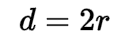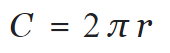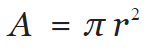Request a Tool

Circumference Calculator

Circumference Calculator use to calculate circumference, diameter and area of a circle.

Diameter
0

Formula• d = Diameter of the circle
• r = Radius of the circle

Defination / Uses

Any straight line segment that goes through the centre of the circle and whose endpoints are on the circle is called a diameter of a circle in geometry. It can also be described as the circle's longest chord. Both definitions are correct for a sphere's diameter.

Circumference
0

Formula• π = A constant value 3.1415
• C = Circumference
• r = Radius of the circle

Defination / Uses

The circumference of a circle or ellipse is defined as its perimeter in geometry. As if the circle were expanded up and smoothed out to a line segment, the circumference would be the arc length of the circle. The perimeter of any closed figure is the length of the arc around it.

Area
0

Formula• π = A constant value 3.1415
• A = Area of the circle
• r = Radius of the circle

Defination / Uses

The area contained by a circle of radius r in geometry is πr². The Greek letter represents the constant ratio of a circle's circumference to its diameter, which is roughly equal to 3.1416.

The circumference of a circle is the length of the circle's circumference. It's comparable to the perimeter of other shapes, such as squares. You can think of it as the shape's defining line. The perimeter is the defining line for objects with straight edges, whereas the circumference is the defining line for circles. The radius (r) and the diameter (d) are two more crucial distances on a circle (d). Every circle has three defining features: a radius, a diameter, and a circumference. The circumference can be calculated using the radius or diameter and pi. The diameter of a circle is the distance between one side and the other at its widest points.